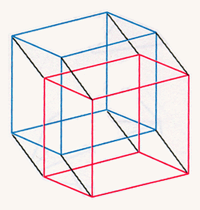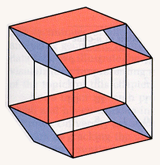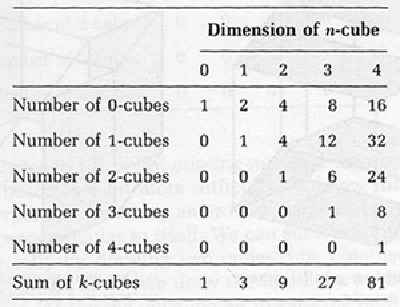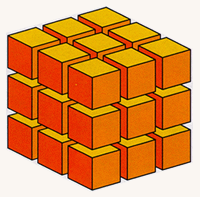Counting the Faces of Higher-Dimensional Cubes

Analogous to the sequence of simplexes in each dimension, we have a sequence of cubes. We begin a table.Moving a cube perpendicular to itself creates a hypercube.

When we try to fill in the missing numbers for a hypercube, the process becomes a bit more difficult. We know that we can generate a hypercube by taking an ordinary cube and moving it in a direction perpendicular to itself. We can show what is happening schematically by drawing two cubes, one obtained by displacement from the other. We draw the first cube in red and the second in blue. As the red cube moves toward the blue cube, the 8 vertices trace out 8 parallel edges. We have 12 edges on the red cube, 12 on the blue, and now 8 new edges for a total of 32 edges on the hypercube.

Finding the number of square faces on the hypercube presents more of a problem, but a version of the same method can solve it. There are 6 squares on the red cube and 6 on the blue one, and we also find 12 squares traced out by the edges of the moving cube for a total of 24.

The edges in the hypercube come in four groups of 8 parallel edges. Similarly the squares can be considered as six groups of 4 parallel squares, one such square through each vertex. The illustration on the bottom, left, shows two groups of 4 parallel squares. Another group is shown on the right. We can then go on to identify the remaining three groups of 4 squares to obtain the entire set of 24 squares in the hypercube. Note that it is easier to identify the 4 squares when they do not overlap and relatively more difficult when the overlap is large.Left: Two groups of four parallel square faces in a hypercube. Right: One more group of four parallel faces in a hypercube.

This manner of grouping the faces of an object is particularly effective when the object possesses a great deal of symmetry, as does the hypercube. A segment possesses one symmetry, obtained by interchanging its endpoints. A square has a much larger number of symmetries: we can rotate the square into itself by one, two, or three quarter-turns about its center, and we can reflect the square across either of its diagonals, or across the horizontal or vertical lines through its center. The even larger group of symmetries of the cube enables us to move any vertex to any other vertex and any edge and square at that vertex to a chosen edge and square at the new vertex. The collection of symmetries is one of the most important examples of an algebraic structure known as a group. The analysis of symmetry groups has provided extremely significant tools in modern geometry and in the applications of geometry to molecular chemistry and quantum physics.

The hypercube is so highly symmetric that every vertex looks like every other vertex. If we know what happens at one vertex, we can figure out what is going to happen at all vertices. At each vertex there are as many square faces as there are ways to choose 2 edges from among the 4 edges at the point, namely 6. Since there are 16 vertices, we can multiply 6 by 16 to get 96, but this counts each square four times, once for each of its vertices. The correct number of squares in a hypercube is then 96/4, or 24.

It is possible to express these results in a general formula. Let Q(k, n) denote the number of k-cubes in an n-cube. To calculate Q(k, n) we may first find out how many k-cubes there are at each vertex. There are n edges emdnating from each vertex, and we get a k-cube for any subset of k distinct edges from among these n edges. Therefore the number of k-cubes at each vertex of an n-cube is C(k, n) = n!/[k!(n - k)!], the combinations of n things taken k at a time. Since we have C(k, n) k-cubes at each of the 2n vertices, we obtain a total number 2nC(k, n). But in this count, each k-cube is counted 2k times, so we divide by that number to get the final formula: Q(k, n) = 2n-kC(k, n) .

An inspection of the table reveals that the entries in each column add up to a power of 3.There are several ways to verify this observation. We can observe that each entry is the sum of twice the entry directly to the left of it plus the entry above that one, so the sum of entries in one column is three times the sum of the entries in the previous column. This argument can easily be translated into a formal proof by mathematical induction. We may also use the explicit formula for the number of k-cubes in an n-cube and observe the sumA cube subdivided into 27 smaller cubes.

Q(0,n) + Q(1,n) + ... + Q(n-1,n) + Q(n,n)
= 2n + C(1,n)2n-1 + C(2,n)2n-2 + ... + C(n-1,n)2 + C(n,n)
= (2 + 1)n = 3n

The most satisfying demonstration of this algebraic fact comes from the observation that we may divide the sides of an n-cube into three parts and extend this to divide the entire cube into 3n small cubes. We then have a small cube coming from each of the vertices of the original, and one from each edge and one from each two-dimensional face, and so on. The final small cube is in the center. Thus the total number of small cubes is the sum of the numbers of k-cubes in the n-cube, and this value is 3n.

One of Friedrich Froebel's kindergarten gifts was a cube subdivided into 27 small cubes. He would have liked this final demonstration.